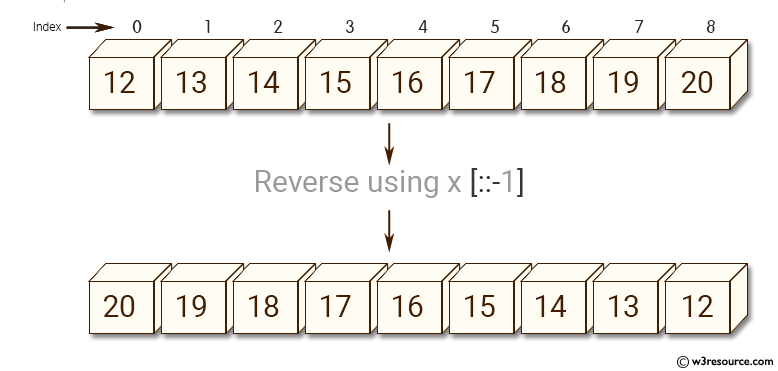﻿ NumPy: Reverse an array - w3resource# NumPy: Reverse an array

## NumPy: Array Object Exercise-6 with Solution

Write a NumPy program to reverse an array (first element becomes last).Sample Solution:-

Python Code:

``````import numpy as np
import numpy as np
x = np.arange(12, 38)
print("Original array:")
print(x)
print("Reverse array:")
x = x[::-1]
print(x)
```
```

Sample Output:

```Original array:
[12 13 14 15 16 17 18 19 20 21 22 23 24 25 26 27 28 29 30 31 32 33 34 35
36
37]
Reverse array:
[37 36 35 34 33 32 31 30 29 28 27 26 25 24 23 22 21 20 19 18 17 16 15 14
13
12]
```

Python Code Editor:

Have another way to solve this solution? Contribute your code (and comments) through Disqus.

What is the difficulty level of this exercise?

Test your Python skills with w3resource's quiz

﻿

## Python: Tips of the Day

While-else construct:

```i = 5

while i > 1:
print("Whil-ing away!")
i -= 1
if i == 3:
break
else:
print("Finished up!")
```